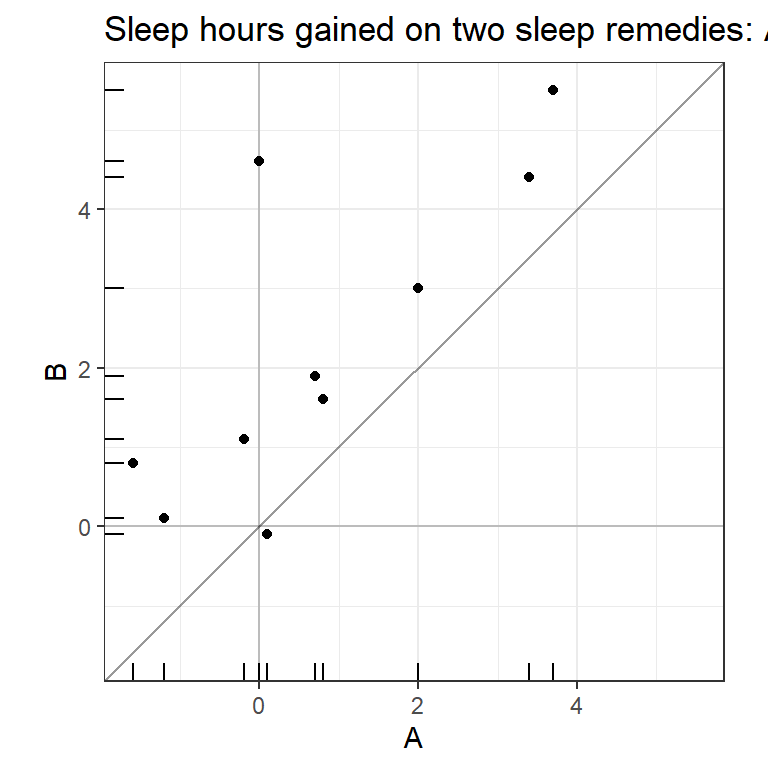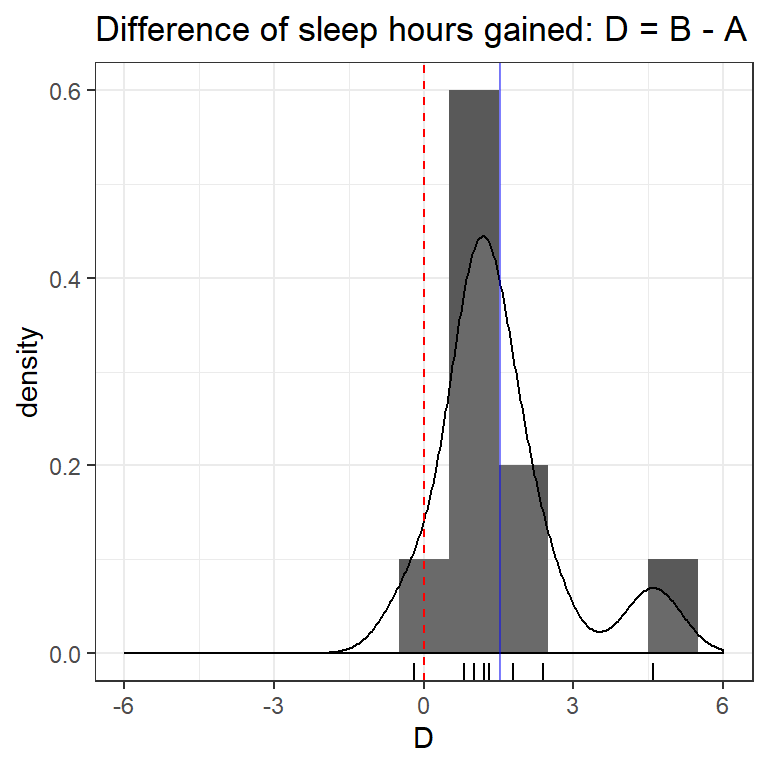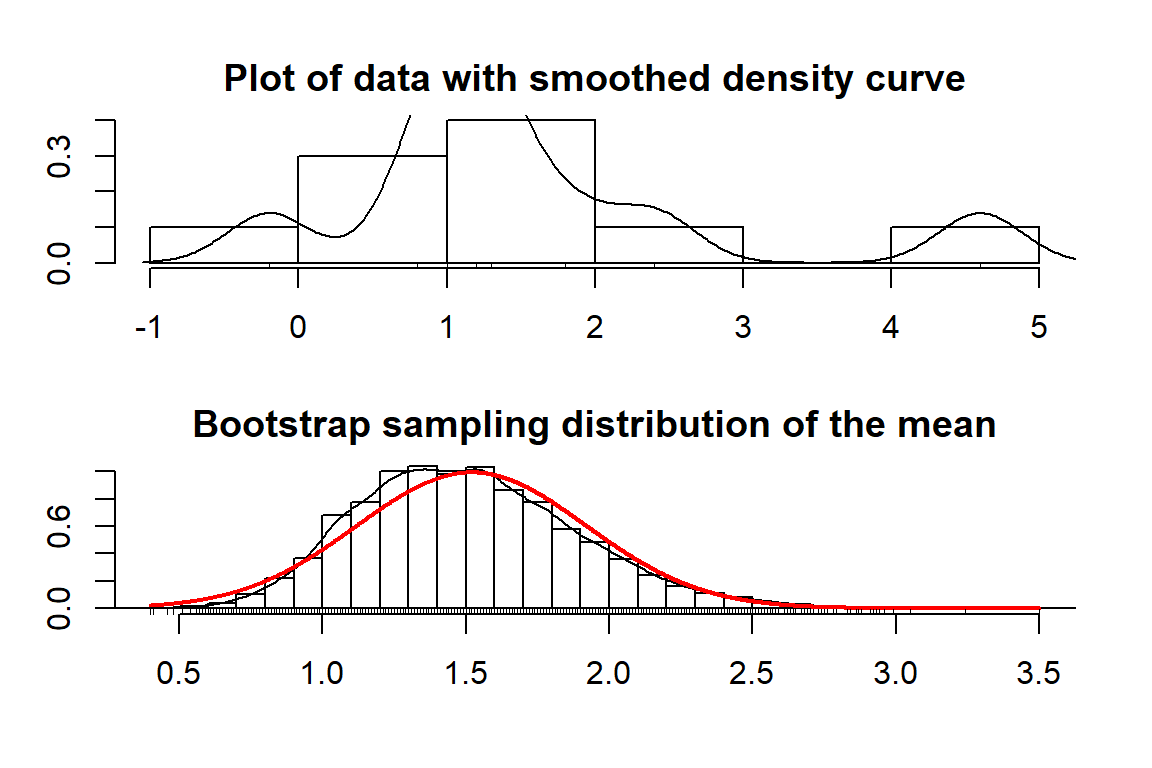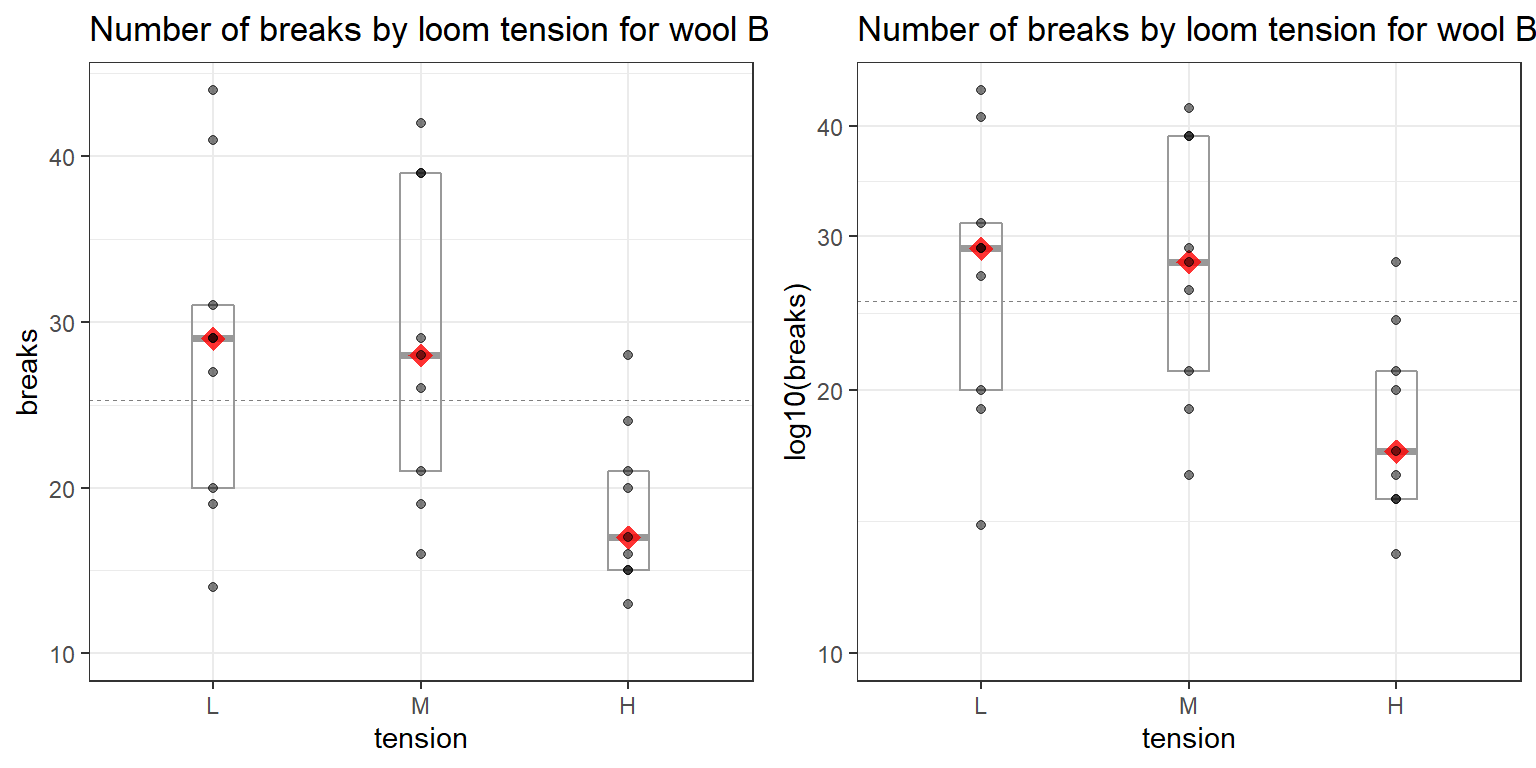Include your answers in this document in the sections below the rubric where I have point values indicated (1 p).

# Rubric

Answer the questions with the data examples.

library(tidyverse)
## -- Attaching packages --------- tidyverse 1.2.1 --
## v ggplot2 3.2.1     v purrr   0.3.2
## v tibble  2.1.3     v dplyr   0.8.3
## v tidyr   1.0.0     v stringr 1.4.0
## v readr   1.3.1     v forcats 0.4.0
## -- Conflicts ------------ tidyverse_conflicts() --
## x dplyr::lag()    masks stats::lag()

# Paired Comparisons of Two Sleep Remedies, revisited

We revisit the sleep remedy dataset (from WS16) because the normality assumption of the sampling distribution of the mean did not seem to be reasonable. The plots below remind us of the data and bootstrap estimate of the sampling distribution.## Warning: Removed 2 rows containing missing values (geom_bar).Use the example in ADA1 Chapter 6 on Nonparametric Methods to state the hypothesis and results of the tests:

## Sign test

(1 p) Hypotheses in words and notation. Be sure to specify which population parameter is being tested.

(1 p) R code and results for test (use the BSDA package and SIGN.test()). Use $$\alpha = 0.10$$.

(1 p) Conclusion based on test.

## 90% CI for the Median

(0.5 p) R code and CI (use the wilcox.test()).

(0.5 p) State the assumptions for the Wilcoxon CI.

(1 p) Determine the appropriate method for a CI (sign or Wilcoxon), then present and interpret the 90% CI with reference to the population parameter.

# The Number of Breaks in Yarn during Weaving

This data set gives the number of warp breaks per loom, where a loom corresponds to a fixed length of yarn. We will analyze wool type B and see whether the tension affects the number of breaks.

A data frame with 54 observations on 3 variables.
[,1]  breaks  numeric The number of breaks
[,2]  wool    factor  The type of wool (A or B)
[,3]  tension factor  The level of tension (L, M, H)
library(datasets)
str(warpbreaks)
## 'data.frame':    54 obs. of  3 variables:
##  $breaks : num 26 30 54 25 70 52 51 26 67 18 ... ##$ wool   : Factor w/ 2 levels "A","B": 1 1 1 1 1 1 1 1 1 1 ...
##  $tension: Factor w/ 3 levels "L","M","H": 1 1 1 1 1 1 1 1 1 2 ... # keep only wool type B, then drop wool type from data frame warp.sub <- subset(warpbreaks, wool == "B", select = -wool) str(warp.sub) ## 'data.frame': 27 obs. of 2 variables: ##$ breaks : num  27 14 29 19 29 31 41 20 44 42 ...
##  $tension: Factor w/ 3 levels "L","M","H": 1 1 1 1 1 1 1 1 1 2 ... Notice that in the plot below I include the points, median, and IQR (the center 50%). Because there’s only 9 observations per group the boxplot and violin plot that I would typically also include becomes distracting and less informative. With very small sample sizes, the salience of individual values should increase relative to summaries. # A set of useful summary functions is provided from the Hmisc package: library(Hmisc) ## Loading required package: lattice ## Loading required package: survival ## Loading required package: Formula ## ## Attaching package: 'Hmisc' ## The following objects are masked from 'package:dplyr': ## ## src, summarize ## The following objects are masked from 'package:base': ## ## format.pval, units stat_sum_df <- function(fun, geom="crossbar", ...) { stat_summary(fun.data=fun, colour="gray60", geom=geom, width=0.2, ...) } # Plot the data using ggplot library(ggplot2) p <- ggplot(warp.sub, aes(x = tension, y = breaks)) # plot a reference line for the global mean (assuming no groups) p <- p + geom_hline(yintercept = mean(warp.sub$breaks),
colour = "black", linetype = "dashed", size = 0.3, alpha = 0.5)
# box for IQR and median
p <- p + stat_sum_df("median_hilow", fun.args = list(conf.int=0.5), show.legend=FALSE)
# diamond at mean for each group
p <- p + stat_summary(fun.y = median, geom = "point", shape = 18, size = 4,
colour = "red", alpha = 0.8)
# points for observed data
p <- p + geom_point(alpha = 0.5) #position = position_jitter(w = 0.05, h = 0), alpha = 1)
p <- p + labs(title = "Number of breaks by loom tension for wool B")
p <- p + expand_limits(y = 10)
p <- p + theme_bw()
#print(p)

# log scale
p2 <- p
p2 <- p2 + scale_y_log10(breaks = seq(0, 50, by=10))
p2 <- p2 + labs(y  = "log10(breaks)")
p2 <- p2 + expand_limits(y = 10)
#print(p2)

library(gridExtra)
##
## Attaching package: 'gridExtra'
## The following object is masked from 'package:dplyr':
##
##     combine
grid.arrange(p, p2, ncol=2)Some numerical summaries may be helpful later.

# summary of each
by(warp.sub$breaks, warp.sub$tension, summary)
## warp.sub$tension: L ## Min. 1st Qu. Median Mean 3rd Qu. Max. ## 14.00 20.00 29.00 28.22 31.00 44.00 ## -------------------------------------------------------- ## warp.sub$tension: M
##    Min. 1st Qu.  Median    Mean 3rd Qu.    Max.
##   16.00   21.00   28.00   28.78   39.00   42.00
## --------------------------------------------------------
## warp.sub$tension: H ## Min. 1st Qu. Median Mean 3rd Qu. Max. ## 13.00 15.00 17.00 18.78 21.00 28.00 # IQR, sd, and n by(warp.sub$breaks, warp.sub$tension, function(X) { c(IQR(X), sd(X), length(X)) }) ## warp.sub$tension: L
##  11.000000  9.858724  9.000000
## --------------------------------------------------------
## warp.sub$tension: M ##  18.000000 9.431036 9.000000 ## -------------------------------------------------------- ## warp.sub$tension: H
##  6.000000 4.893306 9.000000

Nonparametric methods are available to use even when assumptions for other tests are met. Use the Kruskal-Wallis rank sum test to assess whether there are differences in medians between these three groups. If there are, determine which pairs differ using the Wilcox-Mann-Whitney test.

(1 p) State the hypothesis.

Perform the KW test.

# KW ANOVA
fit.bt <- kruskal.test(breaks ~ tension, data = warp.sub)
fit.bt
##
##  Kruskal-Wallis rank sum test
##
## data:  breaks by tension
## Kruskal-Wallis chi-squared = 6.905, df = 2, p-value = 0.03167

(1 p) State the assumptions of the KW test and provide the support that the assumptions are either met or not.

(1 p) Interpret the test result above. Use $$\alpha = 0.10$$.

Follow-up pairwise comparisons.

wilcox.test(breaks ~ tension, data = warp.sub, subset = (tension %in% c("L", "M")))
## Warning in wilcox.test.default(x = c(27, 14, 29, 19, 29, 31, 41, 20, 44), :
## cannot compute exact p-value with ties
##
##  Wilcoxon rank sum test with continuity correction
##
## data:  breaks by tension
## W = 41.5, p-value = 0.9647
## alternative hypothesis: true location shift is not equal to 0
wilcox.test(breaks ~ tension, data = warp.sub, subset = (tension %in% c("L", "H")))
## Warning in wilcox.test.default(x = c(27, 14, 29, 19, 29, 31, 41, 20, 44), :
## cannot compute exact p-value with ties
##
##  Wilcoxon rank sum test with continuity correction
##
## data:  breaks by tension
## W = 64.5, p-value = 0.03768
## alternative hypothesis: true location shift is not equal to 0
wilcox.test(breaks ~ tension, data = warp.sub, subset = (tension %in% c("M", "H")))
## Warning in wilcox.test.default(x = c(42, 26, 19, 16, 39, 28, 21, 39, 29), :
## cannot compute exact p-value with ties
##
##  Wilcoxon rank sum test with continuity correction
##
## data:  breaks by tension
## W = 67.5, p-value = 0.01897
## alternative hypothesis: true location shift is not equal to 0

(1 p) Interpret the pairwise comparisons above (which pairs differ) at a Bonferroni-corrected significance level of $$\alpha/3 = 0.0333$$.

(1 p) Summarize results by ordering the means and grouping pairs that do not differ (see notes for examples).

REPLACE THIS EXAMPLE WITH YOUR RESULTS.

Example:  Groups A and C differ, but B is not different from either.
(These groups are ordered by mean, so A has the lowest median and C has the highest.)

Group A   Group B   Group C
-----------------
-----------------